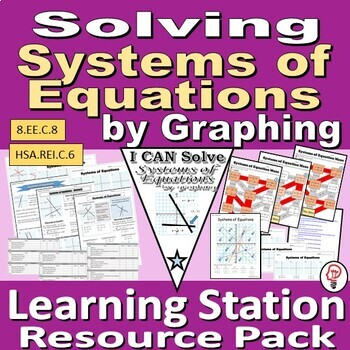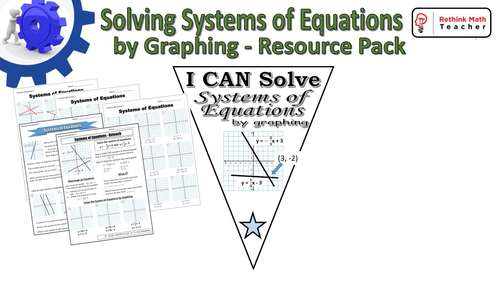# Solve Systems of Equations by Graphing - Learning Stations Resource PackSubject
Resource Type
File Type

Zip

(2 MB|20 pages)
Product Rating
Standards
• Product Description
• StandardsNEW

All the resources you need to create a learning station for Solving Systems of Equations by Graphing.

This resource pack is designed to help you set up a learning station that provides students with quality instruction, lots of practice, and immediate feedback.

Resource Pack includes:

• 3 Reteach Worksheets
• These worksheets are designed to teach the student the skill without the need of direct instruction. Critical thinking skills and guided practice are utilized within each worksheet. Preview the Reteach Worksheets here

• 4 Maze Worksheets
• A fun way to practice the skill while still getting immediate feedback. Each worksheet guides students through a maze by correctly answering the skill. Preview the maze worksheets here.

• 3 Student Tracking Forms
• These are designed to help the student know which activity to do next as they progress through the activities to obtain mastery. One is filled out with online links, and one utilizes only the resources in this packet in case you do not have access to technology in the classroom.

• Pennants to Celebrate Student Success
• One is in color, the other is black and white so that students can fill out

• A document with 10 links to online tutorial videos
• These are links that you can send the student to for help with their reteach, or use in the classroom as reinforcement

• 3 Quizzes
• Short, 5 question quizzes to measure whether or not your student has mastered the skill and is ready to be progressed. If they have not, they can move on to the next set of activities on the skill (provided).

To preview all resources, watch the Video Preview

This resource is part of an Integers Resource Pack Bundle that also includes adding integers, subtracting integers, and. multiplying and dividing integers. Preview the Bunde here.

Solve systems of linear equations exactly and approximately (e.g., with graphs), focusing on pairs of linear equations in two variables.
Solve systems of two linear equations in two variables algebraically, and estimate solutions by graphing the equations. Solve simple cases by inspection. For example, 3𝘹 + 2𝘺 = 5 and 3𝘹 + 2𝘺 = 6 have no solution because 3𝘹 + 2𝘺 cannot simultaneously be 5 and 6.
Understand that solutions to a system of two linear equations in two variables correspond to points of intersection of their graphs, because points of intersection satisfy both equations simultaneously.
Analyze and solve pairs of simultaneous linear equations.
Total Pages
20 pages
Included
Teaching Duration
N/A
Report this Resource to TpT
Reported resources will be reviewed by our team. Report this resource to let us know if this resource violates TpT’s content guidelines.